Next: 5.2 Red-Black Trees Up: 5.1 AVL Trees Previous: 5.1.1 Maximum Height of an AVL Tree

## 5.1.2 AVL Trees: Insertions and Deletions

• While inserting a new node or deleting an existing node, the resulting tree may violate the (stringent) AVL property. To reinstate the AVL property, we use rotations. See Figure 5.4.

Rotation in a BST

• Left rotation and right rotation can be realized by a three-way rotation of pointers.

• Left Rotation:  temp = p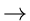right ; pright = templeft ; templeft = p ; p = temp ;

• Left rotation and right rotation preserve
*
BST property
*
Inorder ordering of keys

Problem Scenarios in AVL Tree Insertions

• left subtree of node has degree higher by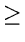2
• left child of node is left high (A)
• left child or node is right high (B)
• right subtree has degree higher by2
• right child of node is left high (C)
• right child or node is right high (D)

• The AVL tree property may be violated at any node, not necessarily the root. Fixing the AVL property involves doing a series of single or double rotations.

• Double rotation involves a left rotation followed or preceded by a right rotation.

• In an AVL tree of height h, no more than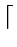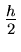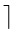rotations are required to fix the AVL property.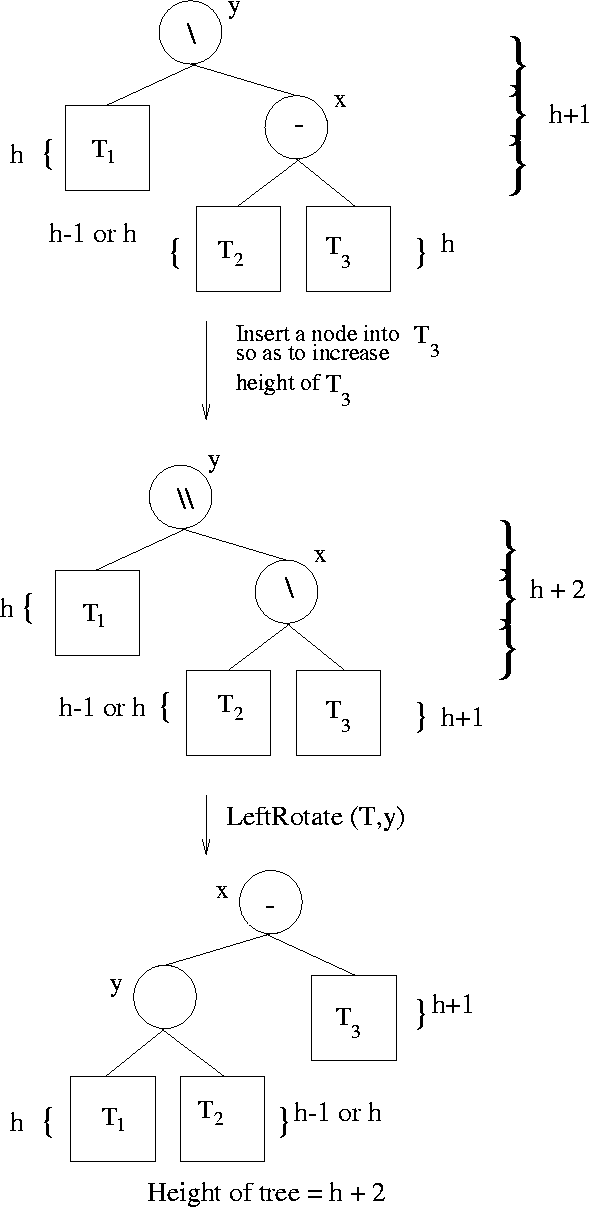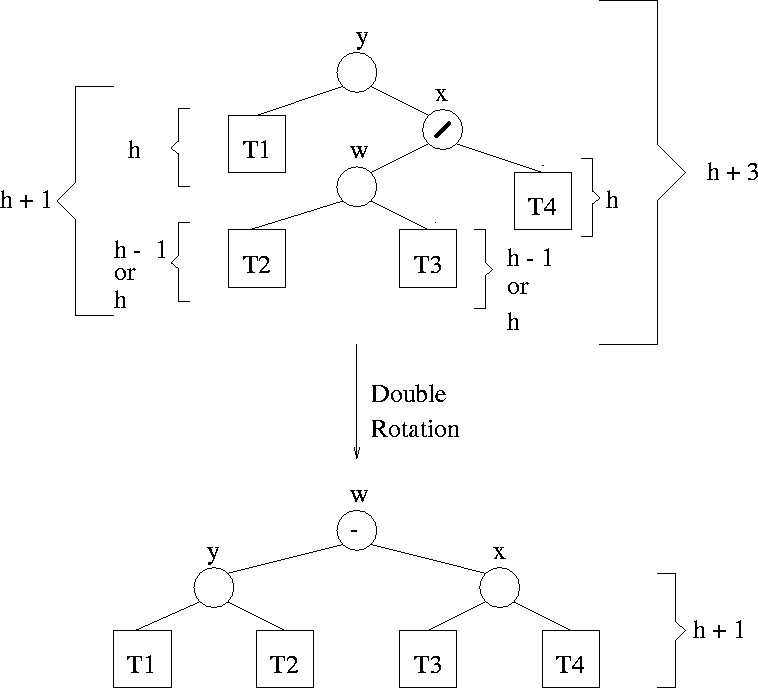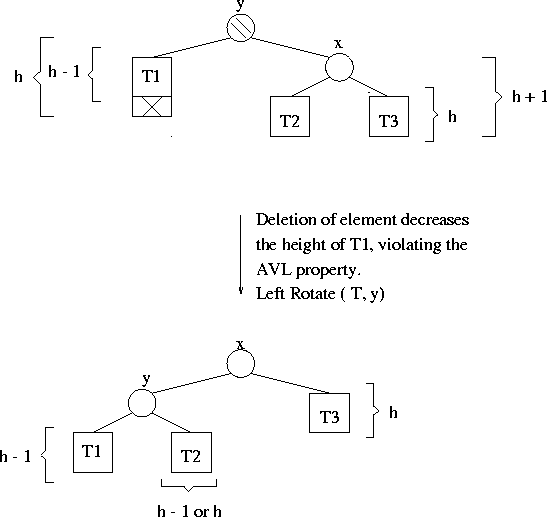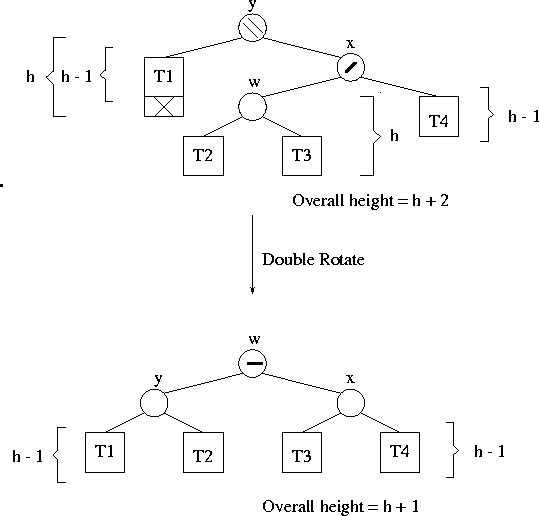Insertion: Problem Scenario 1: (Scenario D)

Scenario A is symmetrical to the above. See Figure 5.5.

Insertion: Problem Scenario 2: (Scenario C)

Scenario B is symmetrical to this. See Figure 5.6.

Deletion: Problem Scenario 1:

Depending on the original height of T2, the height of the tree will be either unchanged (height of T2 = h) or gets reduced (if height of T2 = h - 1). See Figure 5.7.
There is a scenario symmetric to this.

Deletion: Problem Scenario 2:

See Figure 5.8. As usual, there is a symmetric scenario.Next: 5.2 Red-Black Trees Up: 5.1 AVL Trees Previous: 5.1.1 Maximum Height of an AVL Tree
eEL,CSA_Dept,IISc,Bangalore